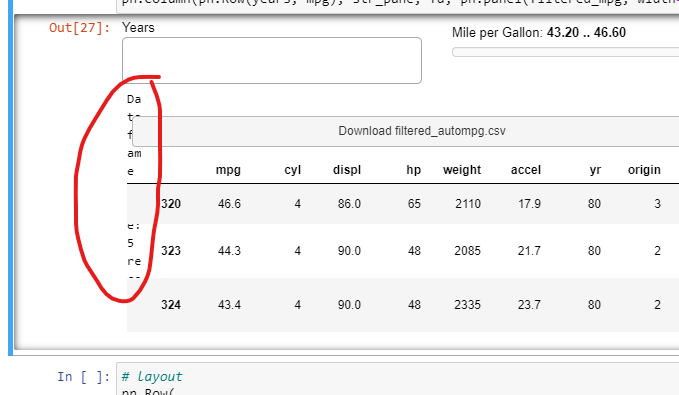# Save filtered DF example: How to return the size of the DF also?

I am following along with the examples, and am currently on the “Save Filtered DF” one here: https://panel.holoviz.org/gallery/simple/save_filtered_df.html#simple-gallery-save-filtered-df

I would like to add an extra line that specifies the size of the filtered dataframe. I’ll just have a line of text saying “Filtered size: 2,000 records”. For this I’ll need to simply get `len(df)`.

Now , I can’t figure out how to get the `len(df)` back from the `filtered mpg` function!

``````@pn.depends(years, mpg)
def filtered_mpg(yrs, mpg):
df = autompg
if years.value:
df = autompg[autompg.yr.isin(yrs)]
return df[(df.mpg >= mpg) & (df.mpg <= mpg)]
``````

Ideally I would like to get the number of records here. I have tried:

``````return df[(df.mpg >= mpg) & (df.mpg <= mpg)] , len(df[(df.mpg >= mpg) & (df.mpg <= mpg)])
``````

I have also tried returning both of them in a list which I was hoping to index:

``````return [df[(df.mpg >= mpg) & (df.mpg <= mpg)] , len(df[(df.mpg >= mpg) & (df.mpg <= mpg)])]

``````

I just need the size of the DF to put it here in the final line:

``````pn.Column(pn.Row(years, mpg), **text_stating_size_of_df**, fd, pn.panel(filtered_mpg, width=600), width=600).servable()
``````

I came up with a very inefficient solution. I replicated the `filtered_mpg` function, and made it only return a string with the size. But now there are two almost duplicate functions working on the same `df`.

``````@pn.depends(years, mpg)
def filtered_mpg_size(yrs, mpg):
df = autompg
if years.value:
df = autompg[autompg.yr.isin(yrs)]
size_of_df = len(df[(df.mpg >= mpg) & (df.mpg <= mpg)])
return f'Dataframe size: {size_of_df} records'
``````

This works … but surely there is a more efficient or logical way. I am not a computer programmer so I think I’ve reached the limits of my “logic” thinking.

@pipo123

See this minimal modification to the example you referenced for one way to accomplish. I added two lines that create a panel Str pane and update its text when you invoke the file save.

``````import panel as pn

from bokeh.sampledata.autompg import autompg
from io import StringIO

pn.extension()

years = pn.widgets.MultiChoice(
name='Years', options=list(autompg.yr.unique()), margin=(0, 20, 0, 0)
)
mpg = pn.widgets.RangeSlider(
name='Mile per Gallon', start=autompg.mpg.min(), end=autompg.mpg.max()
)

@pn.depends(years, mpg)
def filtered_mpg(yrs, mpg):
df = autompg
if years.value:
df = autompg[autompg.yr.isin(yrs)]
return df[(df.mpg >= mpg) & (df.mpg <= mpg)]

@pn.depends(years, mpg)
def filtered_file(yr, mpg):
df = filtered_mpg(yr, mpg)
str_pane.object = 'Dataframe size: {:d} records'.format(len(df))
sio = StringIO()
df.to_csv(sio)
sio.seek(0)
return sio

callback=filtered_file, filename='filtered_autompg.csv'
)

str_pane = pn.pane.Str(object='')

pn.Column(pn.Row(years, mpg), str_pane, fd, pn.panel(filtered_mpg, width=600), width=600).servable()``````
1 Like

Thank you. However is there any way to get the `df` size before clicking the download button? That’s what I wanted to do originally. As in, let the user know that they could be downloading something with 1 million rows. Maybe they will decide to filter a bit more before doing that.

With the above solution I am getting this weird text position also.@pipo123

Try the following, which just reorganizes where the panel `Str` pane is updated.

Also, I do not run panel in a notebook, so I did not experience the mangled text when running in a panel server context. I explicitly set the width of the `Str` pane in the update below to hopefully address what you observe.

``````#!/usr/bin/env python3
# -*- coding: utf-8 -*-
"""
"""

import panel as pn

from bokeh.sampledata.autompg import autompg
from io import StringIO

pn.extension()

years = pn.widgets.MultiChoice(
name='Years', options=list(autompg.yr.unique()), margin=(0, 20, 0, 0)
)
mpg = pn.widgets.RangeSlider(
name='Mile per Gallon', start=autompg.mpg.min(), end=autompg.mpg.max()
)

@pn.depends(years, mpg)
def filtered_mpg(yrs, mpg):
df = autompg
if years.value:
df = autompg[autompg.yr.isin(yrs)]
rv = df[(df.mpg >= mpg) & (df.mpg <= mpg)]
str_pane.object = 'Dataframe size: {:d} records'.format(len(rv))
return rv

@pn.depends(years, mpg)
def filtered_file(yr, mpg):
df = filtered_mpg(yr, mpg)
sio = StringIO()
df.to_csv(sio)
sio.seek(0)
return sio

callback=filtered_file, filename='filtered_autompg.csv'
)

str_pane = pn.pane.Str(object='', width=600)

pn.Column(pn.Row(years, mpg), str_pane, fd, pn.panel(filtered_mpg, width=600), width=600).servable()``````

Hi @pipo123

If you want to have even more control over when the application updates what, you can try using a `param.Parameterized` class like the below.

``````#!/usr/bin/env python3
# -*- coding: utf-8 -*-
"""
"""

import panel as pn
import param

from bokeh.sampledata.autompg import autompg
from io import StringIO

raw_data = param.DataFrame(constant=True)

years = param.List(label="Years")
mpg = param.Tuple(label="Mile per Gallon")

gold_data = param.DataFrame()
gold_data_rows = param.Integer()

view = param.Parameter()

def __init__(self, raw_data, **params):
params["raw_data"] = raw_data
super().__init__(**params)
self._create_view()
self.mpg = (self.raw_data.mpg.min(), self.raw_data.mpg.max())

def _create_view(self):
self.selection_panel = pn.Param(
self,
parameters=["years", "mpg"],
widgets={
"years": {
"type": pn.widgets.MultiChoice,
"options": list(self.raw_data.yr.unique()),
"margin": (0, 50, 0, 0),
"width": 250,
},
"mpg": {
"type": pn.widgets.RangeSlider,
"start": self.raw_data.mpg.min(),
"end": self.raw_data.mpg.max(),
"width": 300,
},
},
show_name=False,
default_layout=pn.Row,
)
self.str_panel = pn.pane.Str(object="", width=700)

callback=self._get_gold_data_as_file, filename="filtered_autompg.csv"
)

self.table_panel = pn.pane.DataFrame(height=200, width=700, sizing_mode="fixed")

self.view = pn.Column(
self.selection_panel,
self.str_panel,
self.table_panel,
width=700,
)

def _get_gold_data_as_file(self):
df = self.gold_data
sio = StringIO()
df.to_csv(sio)
sio.seek(0)
return sio

@param.depends("years", "mpg", watch=True)
def _update_gold_data(self, *events):
years = self.years
mpg = self.mpg
df = self.raw_data

if years:
df = df[df.yr.isin(years)]
self.gold_data = df[(df.mpg >= mpg) & (df.mpg <= mpg)]
self.gold_data_rows = len(self.gold_data)

@param.depends("gold_data_rows", watch=True)
def _update_str_panel(self, *events):
self.str_panel.object = f"Rows: {self.gold_data_rows}"

@param.depends("gold_data", watch=True)
def _update_table_panel(self, *events):
self.table_panel.object = self.gold_data